# Introduction and models used

In the real problem, there is the presence of three phases : air, oil and water. The study of multiphase flow is often complex. It's why, tackling directly the three phase-flow resolution could be very difficult and could lead to a misunderstanding of the simplest principles of the problem. Then  we decided, first of all to begin our study with simple cases and studying them for different parameters combinations.

In the one phase flow case we consider that there is only one fluid presents in the whole domain of resolution and we resolve the simple conservation equation in which Darcy law is injected.

We used the following equations :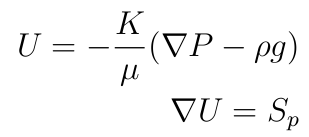where :

$U$ :  the velocity field

$P$ : the pressure

$S_p$ :  the source term

$K$ : the permeability

$g$ : the gravity

$\rho$ : the density

$\mu$ : the dynamic viscosity

In some studies the gravity will be neglected.

To resolve the conservation equation in which Darcy law is injected  we developed a solver that we called a Darcy solver or darcyFoam by modifying the existing laplacianFoam solver in OpenFoam solver directory. Then we modified it in order to compute the pressure field and having Darcy velocity.

After developing this simple Darcy solver, we added a source term that corresponds to the rate flow in the extractor well.

The second step was the definition of the permeability, no more as a simple scalar but as  a scalar field or as a tensor.

Finally we added to our solver the gravity, which  needed a real understanding of the gravity influence on boundaries conditions of the domain of study. Indeed the gravity introduction leads to a new boundary condition on the pressure gradient on the bottom of the domain and the inversion of the permeability tensor which is complex to program on OpenFoam. The boundary condition on the bottom will be expressed by :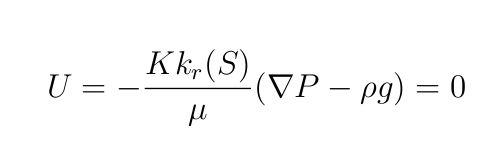On OpenFoam  we used flux through cells surface to set this boundary condition, then the condition of zero velocity on the domain bottom is transformed to a condition of zero flux.

The flux is expressed by :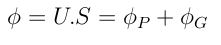S is the cell surface vector, where :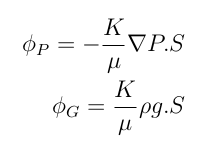Moreover $U=0$ leads to $\phi=0$,  then :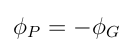Finally we have the following boundary condition on the pressure gradient :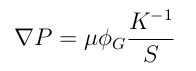To avoid having a matrix inversion, the permeability K was defined as a simple scalar or as a field of scalar as a first approach.

When the permeability is defined as a scalar or as a scalar field have :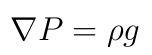In this parts we will study the influence of the anisotropy, the gravity and the flow rate on the flow to try different parameters and to study the stability of the solver.

In all the studies of this part, the fluid has the following physical properties :

$\rho=1000 \ Kg/m^3$

$\mu=6,4 \ 10^{-4} \ Kg/(m.s)$

Those properties are almost the properties of petrol.

Moreover the flow rate  called qwell will be fixed to :

$qwell=1 \ m^3/s$.

# Influence of the anisotropy

As we know the large majority of porous media that we can find in industry or in the nature present an anisotropy. It's why it is interesting to understand the influence of the anisotropy on the flow.

In order to take into account the anisotropy, we define the permeability as a tensor field in $m^2$:First of all we study a case with K defined as a tensor but without anisotropy in $m^2$:Then we can notice, that in the anisotropic K introduced, the permeability is more important in the horizontal direction than in the vertical or perpendicularly direction. Which means that we should have a more important velocity in the horizontal direction.

In the following study the gravity influence was neglected.

# Influence of the anisotropy on the velocity field

Velocity field in anisotropic case :Velocity field in isotropic case :We observe that in the anisotropic case the velocity magnitude is more important in the horizontal direction than in the vertical one as it was predicted in theory. Indeed this result is normal because the permeability is more important in the horizontal direction than in the other directions.

# Influence of the anisotropy on the pressure field

Pressure field in the case of isotropy :Pressure field in the case of anisotropy :As we have decreased the permeability in the vertical direction in the anisotropic case, the velocity is decreased which increases the pressure.

# Gravity influence

Taking into account the gravity in the darcyFoarm solver, is complex and needs a good basics in programming on OpenFoam.

Indeed having a gravity field involves a new boundary condition in the bottom of the domain on the pressure gradient.
Then the condition zero velocity on the bottom is expressed by :then we have the following boundary condition on the pressure gradient:After adding the gravity to the solver, we decided to compare the case with gravity to the case without gravity term that intervenes in Darcy law.
In this study the solver used is a solver without anisotropy, moreover the permeability will be equal to $K=7.10 {-7} \ m^2$.

Therefore we studied the influence of the gravity on the pressure and on the velocity fields :

# Gravity influence on the velocity field

Velocity field without gravity presence :Velocity field with gravity presence :We notice that the gravity has few impact on the velocity field.

# Gravity influence on the pressure field

Pressure without gravity field :Pressure with gravity field :We notice that the gravity decreases the negative maximum pressure.

# Well size influence

As on the field, a well implementation is hugely expensive and a mistake could lead to huge economical losses. It's important to know the best dimensions and the most adapted to the problem before drilling.

We tested in this part three well dimensions. And we studied the behavior of the flow as a function of the well diameter that we have called D.

The solver used is a solver without anisotropy, moreover the permeability will be equal to $K=7.10 {-7} \ m^2$.

# Influence of the well size on the velocity field

Velocity field D=0.2 mVelocity field D=0.5 mVelocicty field  D=1 mWe observe that an increasing in the well diameter  leads to a decreasing of the maximum velocity magnitud.

# Influence of the well size on the pressure field

Pressure field D=0.2 mPressure field D= 0.5 mPressure field D= 1 mWe observe that an increasing in the well diameter leads to a increasing of the maximum negative pressure.

# Influence of the flow rate

In this part, we will study the influence of the source term on the flow. The source term corresponds to the flow rate in the well. We call the flow rate qwell.

The study will be led with the parameters presented on the previous parts. The only parameters that will change is the flow rate. The solver used is a solver without anisotropy, moreover the permeability will be equal to $K=7.10 {-7} \ m^2$.

In some cases with very high rate flow the solutions obtained are not real because the Darcy low is valid only for small velocities.

# Influence of the flow rate on the velocity

Velocity field for the case : $qwell=0.5 \ m^3/s$Velocity field for the case : $qwell=1 \ m^3/s$Velocity field for the case : $qwell=10 \ m^3/s$An increasing of the flow rate induce an increasing in the velocity magnitude.

# Influence of the flow rate on the pressure field

Pressure field for the case : $qwell=0.5 \ m^3/s$Pressure field for the case : $qwell=1\ m^3/s$Pressure field for the case : $qwell=10 \ m^3/s$An increase in flow rate induce an increase of the negative pressure.

# Conclusion of monophase flow study

In conclusion, we succeeded to implement the gravity. The presence of the gravity leads to a real impacts on the flow.

It's important to take into account the anisotropy of the domain to have the best description of the flow.

However when we take into account the gravity the  definition of a boundary condition of zero velocity (zero flux in our solver) the bottom of the domain  leads to the matrix permeability inversion. The matrix inversion on OpenFoam is complex to implement. For this reason in the solver with gravity we defined the permeability as a scalar field or as a scalar.

The determination of the well size depends on the objectives of production fixed by the operator and the parameters of the problem.

Finally, our  simulations obtained by the solvers developed in this part are compatible with the theoretical studies found in the literature or data from real fields.